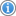# English Language Arts/Literacy and Mathematics Smarter Balanced Summative Assessments

## Detailed Test Results for:District: California Education Authority (CEA) Hea

CDS Code: 34-32276-0000000|County: Sacramento

Report Options

PLEASE NOTE: Achievement level percentages in the same subject can be compared within grade levels, with adjacent grades, and from one year to another. Schools made up of differing grade levels should be compared with caution.

## 2021–22 Detailed Test ResultsResults for All Students

### ENGLISH LANGUAGE ARTS/LITERACY

#### Achievement Level Distribution

In order to protect student privacy, an asterisk (*) will be displayed for enrolled and tested counts for fewer than 4 students and for assessment results for fewer than 11 students. "N/A" will be displayed instead of a number on test results where no data is found for the specific report.

Overall Achievement
Overall Achievement
Number of Students Enrolled This total includes only the students in this student group who were eligible to take the Smarter Balanced test in 2022 in this subject area.N/AN/AN/AN/AN/AN/A2525
Number of Students Tested This total includes only the students who took the Smarter Balanced test in 2022 for this subject area.N/AN/AN/AN/AN/AN/A2222
Number of Students With Scores This total includes only the students who took the Smarter Balanced test in 2022 for this subject area and responded to enough questions to generate a score.N/AN/AN/AN/AN/AN/A2222
Mean Scale Score N/AN/AN/AN/AN/AN/A2382.1N/A
Standard Exceeded: Level 4 The percentage of students at each achievement level is calculated by dividing the number of students at that achievement level by the total number of students with scores in 2022 for the subject area.N/AN/AN/AN/AN/AN/A0.00 %0.00 %
Standard Met: Level 3 The percentage of students at each achievement level is calculated by dividing the number of students at that achievement level by the total number of students with scores in 2022 for the subject area.N/AN/AN/AN/AN/AN/A0.00 %0.00 %
Standard Nearly Met: Level 2 The percentage of students at each achievement level is calculated by dividing the number of students at that achievement level by the total number of students with scores in 2022 for the subject area.N/AN/AN/AN/AN/AN/A4.55 %4.55 %
Standard Not Met: Level 1 The percentage of students at each achievement level is calculated by dividing the number of students at that achievement level by the total number of students with scores in 2022 for the subject area.N/AN/AN/AN/AN/AN/A95.45 %95.45 %

### Areas

Area Achievement Level Descriptors provide a more detailed look at students' performance on the overall assessment. The results in these key areas for each subject are reported using the following three indicators: below standard, near standard, and above standard. The sum of the achievement level percentages may not add to 100% due to rounding.

READING: How well do students understand stories and information that they read?
READING: How well do students understand stories and information that they read?
Above Standard The percentage of students at each claim level is calculated by dividing the number of students at that claim level by the total number of students with scores in 2022 for the subject area.N/AN/AN/AN/AN/AN/A**
Near Standard The percentage of students at each claim level is calculated by dividing the number of students at that claim level by the total number of students with scores in 2022 for the subject area.N/AN/AN/AN/AN/AN/A**
Below Standard The percentage of students at each claim level is calculated by dividing the number of students at that claim level by the total number of students with scores in 2022 for the subject area.N/AN/AN/AN/AN/AN/A**
WRITING: How well do students communicate in writing?
WRITING: How well do students communicate in writing?
Above Standard The percentage of students at each claim level is calculated by dividing the number of students at that claim level by the total number of students with scores in 2022 for the subject area.N/AN/AN/AN/AN/AN/A**
Near Standard The percentage of students at each claim level is calculated by dividing the number of students at that claim level by the total number of students with scores in 2022 for the subject area.N/AN/AN/AN/AN/AN/A**
Below Standard The percentage of students at each claim level is calculated by dividing the number of students at that claim level by the total number of students with scores in 2022 for the subject area.N/AN/AN/AN/AN/AN/A**
LISTENING: How well do students understand spoken information?
LISTENING: How well do students understand spoken information?
Above Standard The percentage of students at each claim level is calculated by dividing the number of students at that claim level by the total number of students with scores in 2022 for the subject area.N/AN/AN/AN/AN/AN/A**
Near Standard The percentage of students at each claim level is calculated by dividing the number of students at that claim level by the total number of students with scores in 2022 for the subject area.N/AN/AN/AN/AN/AN/A**
Below Standard The percentage of students at each claim level is calculated by dividing the number of students at that claim level by the total number of students with scores in 2022 for the subject area.N/AN/AN/AN/AN/AN/A**
Above Standard The percentage of students at each claim level is calculated by dividing the number of students at that claim level by the total number of students with scores in 2022 for the subject area.N/AN/AN/AN/AN/AN/A**
Near Standard The percentage of students at each claim level is calculated by dividing the number of students at that claim level by the total number of students with scores in 2022 for the subject area.N/AN/AN/AN/AN/AN/A**
Below Standard The percentage of students at each claim level is calculated by dividing the number of students at that claim level by the total number of students with scores in 2022 for the subject area.N/AN/AN/AN/AN/AN/A**